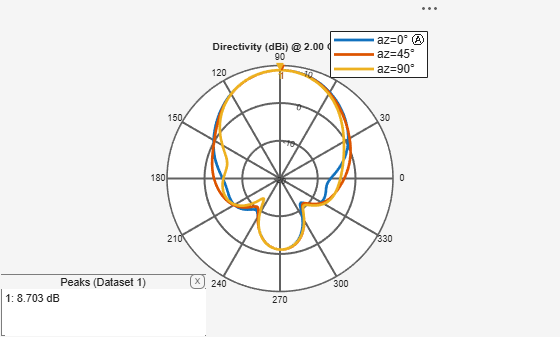## Syntax

``msiread(fname)``
``[horizontal] = msiread(fname)``
``````[horizontal,vertical] = msiread(fname)``````
``````[horizontal,vertical,optional] = msiread(fname)``````

## Description

example

````msiread(fname)` reads an MSI planet antenna file in `.pln`, or `.msi` formats.```
````[horizontal] = msiread(fname)` reads the file and returns a structure containing horizontal gain data. ```
``````[horizontal,vertical] = msiread(fname)``` reads the file and returns structures containing horizontal and vertical gain data. ```

example

``````[horizontal,vertical,optional] = msiread(fname)``` reads the file and returns structures containing horizontal gain data, vertical gain data, and all additional data in the file.```

## Examples

collapse all

Create a helix antenna and plot the elevation pattern at 2 GHz.

```h = helix; patternElevation(h,2e9,[0 45 90],'Elevation',0:1:360);```Write the elevation pattern of the helix antenna in an MSI Planet Antenna file.

`msiwrite(h,2e9,'helix','Name','Helix Antenna Specifications')`

The msiwrite function saves a file named `helix.pln` to the default MATLAB™ folder.

```NAME Helix Antenna Specifications FREQUENCY 2000.0 GAIN 8.74 dBi HORIZONTAL 360 0.00 13.56 1.00 13.48 2.00 13.39 3.00 13.30 4.00 13.22 5.00 13.13 ```

Read the MSI antenna data file created.

`msiread helix.pln`
```ans = struct with fields: PhysicalQuantity: 'Gain' Magnitude: [360x1 double] Units: 'dBi' Azimuth: [360x1 double] Elevation: 0 Frequency: 2.0000e+09 Slice: 'Elevation' ```

Read horizontal, vertical and optional data from the antenna data file Test_file_demo.pln.

`[Horizontal,Vertical,Optional] = msiread('Test_file_demo.pln')`
```Horizontal = struct with fields: PhysicalQuantity: 'Gain' Magnitude: [360x1 double] Units: 'dBd' Azimuth: [360x1 double] Elevation: 0 Frequency: 659000000 Slice: 'Elevation' ```
```Vertical = struct with fields: PhysicalQuantity: 'Gain' Magnitude: [360x1 double] Units: 'dBd' Azimuth: 0 Elevation: [360x1 double] Frequency: 659000000 Slice: 'Azimuth' ```
```Optional = struct with fields: name: 'Sample.pln' make: 'Sample 4DR-16-2HW' frequency: 659000000 h_width: 180 v_width: 7.3000 front_to_back: 34 gain: [1x1 struct] tilt: 'MECHANICAL' polarization: 'POL_H' comment: 'Ch-45 0 deg dt' scaling_mode: 'AUTOMATIC' ```

## Input Arguments

collapse all

Name of MSI file, specified as a character vector. The files must be a `.pln` or `.msi` format.

## Output Arguments

collapse all

Horizontal gain data, returned as a structure containing the following fields:

• `PhysicalQuantity` — Quantity specified in the MSI file, returned as one of the values: `'E-field'`, `'H-field'`, `'directivity'`, `'power'`, `'powerdB'`, or `'Gain'`.

• `Magnitude` — Magnitude values of the quantity specified in the MSI file, returned as a real vector of size `N–by–1` where N is same size as `theta` and `phi` angles.

• `Units` — Units of the quantity specified in the MSI file, returned as one of the values: `'dBi'`, `'dB'`, `'V/m'`, `'watts'`, or `'dBd'`.

• `Azimuth` — Azimuth angles specified in the MSI file, returned as a scalar or a vector in degrees.

• `Elevation` — Elevation angles specified in the MSI file, returned as a scalar or a vector in degrees.

• `Frequency` — Frequency specified in the MSI file, returned as a scalar or a vector in Hertz.

• `Slice` — Type of data set variation, returned as text. The variations are `'Azimuth'` or `'Elevation'`.

Vertical gain data, returned as a structure containing the following fields:

• `PhysicalQuantity` — Quantity specified in the MSI file, returned as one of the values: `'E-field'`, `'H-field'`, `'directivity'`, `'power'`, `'powerdB'`, or `'Gain'`.

• `Magnitude` — Magnitude values of the quantity specified in the MSI file, returned as a real vector of size `N–by–1` where N is same size as `theta` and `phi` angles.

• `Units` — Units of the quantity specified in the MSI file, returned as one of the values: `'dBi'`, `'dB'`, `'V/m'`, `'watts'`, or `'dBd'`.

• `Azimuth` — Azimuth angles specified in the MSI file, returned as a scalar or a vector in degrees.

• `Elevation` — Elevation angles specified in the MSI file, returned as a scalar or a vector in degrees.

• `Frequency` — Frequency specified in the MSI file, returned as a scalar or a vector in Hertz.

• `Slice` — Type of data set variation, returned as text. The variations are `Azimuth` or `Elevation`.

Additional data, returned as a structure containing (but not limited to): `Name`, `Make`, `Frequency`, `H_width`, `V_width`, `Front_to_back`, `Gain`, `Tilt`, `Polarization`, `Comment`.